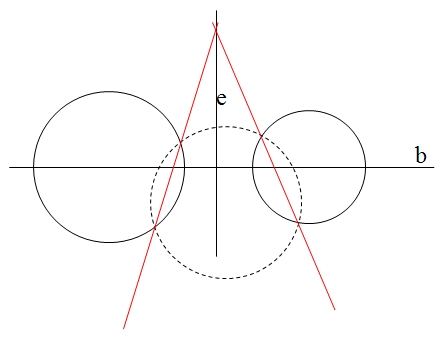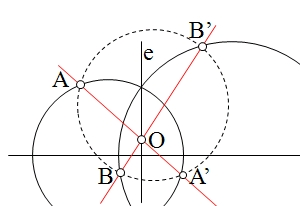# Metric geometry : Obtaining the radical axis of two circles

Is a straight line having a direction perpendicular to the centerline of the circumferences. To determine this axis is therefore necessary to know a single crossing point.

We will see how to determine the radical axis of two circles in the different cases that we can find, to analyze in terms of the relative positions of the two circles.

### Intersecting circles

If the circles are intersecting know two points of zero power, the intersection of the two circles.In this case the radical axis we will get through these common secant circles

### Tangent circles

As in the previous case, there is zero power point corresponding to the nip of the two circles.The radical axis pass through this point and have a direction perpendicular to the line of centers and therefore coincide with the common tangent to both circles.

### Non-intersecting circles

As we know the direction of the axis, will be determined as we get a way point.This point is determine by the use of an auxiliary circle that intersects the two circles, allowing us to obtain the radical center of the three (point of equal power)

As a particular case of interest, We can see the case where circumferences are large radius.In this case is calculated as above, by means of an auxiliary circle (in dashed line in Figure) determining a point “O” equal power with respect to the two circles, as:

OA * OA’ = OB * OB’

This point together with the intersection of the two circles, if any, determine the axis. Otherwise, We could repeat this construction to determine a second crossing point.

It may also be interesting to generalize these concepts in the case of zero radius circles (points) or infinite (straight) and other particular positions:

• Axle? Radical two concentric circles?
• Point? Radical axis and circumference?
• Radical? Point and straight shaft?